## Outline

The vignette covers robust generalized regression (GREG) prediction and robust ratio prediction.

IMPORTANT

It is assumed that the reader is familiar with the key functions of the survey package, like svydesign(), etc. In addition, we assume that the reader has studied the vignette on robust regression. The technical details (incl. references to the literature) of the robust generalized regression and the robust ratio predictor are documented in file /doc/doc_greg.pdf.

## 1 Preparations

First, we load the packages and the MU284pps dataset. The availability of the survey package is imperative.

> library("robsurvey", quietly = TRUE)
> library("survey")
> data("MU284pps")

The MU284pps dataset is random sample from the MU284 population of Särndal et al. (1992, Appendix B). The population includes measurements on the 284 municipalities in Sweden in the late 1970s and early 1980s. It is available in the sampling package; see Tillé and Matei (2021). The sample is a proportional-to-size sample (PPS) without replacement of size 50. The sample has been selected by Brewer’s method; see Tillé (2006, Chap. 7). The sample inclusion probabilities are proportional to the population size in 1975 (variable P75). The sampling weight (inclusion probabilities) are calibrated to the population size and the population total of P75.

The data frame MU284pps includes the following variables.

 LABEL identifier variable P85 1985 population size (in $$10^3$$) P75 1975 population size (in $$10^3$$) RMT85 revenues from the 1985 municipal taxation (in $$10^6$$ kronor) CS82 number of Conservative seats in municipal council SS82 number of Social-Democrat seats in municipal council (1982) S82 total number of seats in municipal council in 1982 ME84 number of municipal employees in 1984 REV84 real estate values in 1984 (in $$10^6$$ kronor) CL cluster indicator REG geographic region indicator weights sampling weights pi finite population correction

First, we define the sampling design

> dn <- svydesign(ids = ~LABEL, fpc = ~pi, data = MU284pps, pps = "brewer")

with the option pps = "Brewer" and the specification that fpc is equal to the first-order sample inclusion probabilities, pi. The design appears (on print) with the following output in the console

> dn
Independent Sampling design
svydesign(ids = ~LABEL, fpc = ~pi, data = MU284pps, pps = "brewer")

The variable of interest is revenues from 1985 taxation (RMT85), and the goal is to estimate the population revenues total (in million Swedish kronor). From register data, the population size in 1985 (variable P85) is a know quantity; it is 8 339 (in thousands). The subsequent graph shows a scatterplot of RMT85 vs. P85 (the size of the circles is proportional to the sampling weight).

> svyplot(RMT85 ~ P85, dn, xlab = "P85", ylab = "RMT85", inches = 0.1)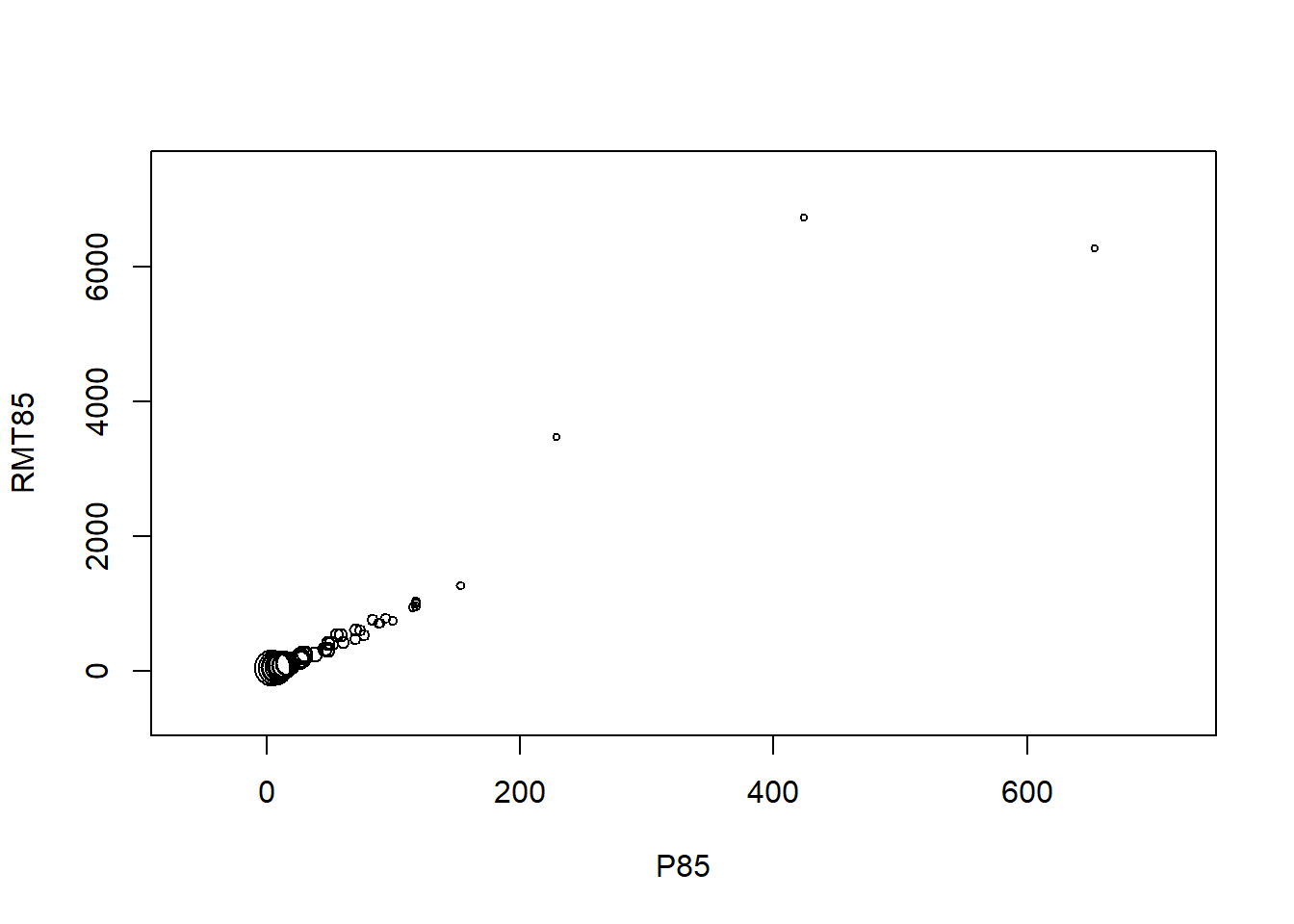## 2 Robust ratio prediction

We are interested in the ratio estimator of the 1985 revenues total. Consider the following population model

$\begin{equation*} \mathrm{RMT85}_i = \mathrm{P85}_i \cdot \theta + \sigma \sqrt{\mathrm{P85}_i} E_i, \qquad i \in U, \end{equation*}$

where the $$E_i$$ are independent and identically distributed random variables with zero mean and unit variance. The parameters $$\theta$$ and $$\sigma > 0$$ are unknown.

#### 2.1 Ratio predictor

Under the model, the least squares (LS) census estimator of $$\theta$$ is $$\theta_N = \sum_{i \in U} y_i / \sum_{i \in U} x_i$$. The sample weighted LS estimator is $$\widehat{\theta}_n = \sum_{i \in s}w_i y_i / \sum_{i \in s} w_i x_i$$, where $$w_i$$ denotes the sampling weight. It is computed by

> rat <- svyratio_huber(~RMT85, ~P85, dn, k = Inf)
> rat

Survey ratio M-estimator (Huber psi, k = Inf)

Call:
svyratio_huber(numerator = ~RMT85, denominator = ~P85, design = dn,
k = Inf)

IRWLS converged in 1 iterations

Coefficients:
RMT85/P85
8.379

Scale estimate: 4.891 (weighted MAD) 

Good to know.

We have used the fact that the Huber $$M$$-estimator of the ratio parameter, svyratio_huber(), with robustness tuning constant k = Inf corresponds to the (non-robust) estimator of the ratio, $$\widehat{\theta}_n$$, introduced above. Following the same train of though, we could have used svyratio_tukey() with k = Inf instead. The robsurvey package does not implement a separate function for the non-robust ratio estimator because the function svyratio() is included in the survey package.

Next, we predict the payroll total based on the estimated regression parameter $$\widehat{\theta}_n$$ (i.e., object rat) and the known population size in 1985 (total = 8339).

> tot <- svytotal_ratio(rat, total = 8339)
> tot
total   SE
RMT85 69872 3214

The estimated mean square error of the ratio predictor is

> mse(tot) / 1e6
 10.33016

which is equal to the estimated variance because the ratio predictor of the total is unbiased for the population total. The estimated total, standard error and variance covariance can be extracted by the functions coef, SE(), and vcov().

#### 2.2 Robust ratio predictor

A robust ratio predictor (with Huber $$\psi$$-function and robustness tuning constant $$k = 20$$) can be computed by

> rat_rob <- svyratio_huber(~RMT85, ~P85, dn, k = 20)
> tot_rob <- svytotal_ratio(rat_rob, total = 8339)
> tot_rob
total   SE
RMT85 68529 2550

In terms of the estimated standard error, this predictor is considerably more efficient than the (non-robust) ratio predictor. We come to the same conclusion if we consider the approximate mean square error

> mse(tot_rob) / 1e6
 8.307178

In place of the Huber estimator, we can use the ratio M-estimator with Tukey biweight (bisquare) $$\psi$$-function; see svyratio_tukey(). The ratio estimator of the population of the mean is computed by svymean_ratio().

## 3 Robust generalized regression prediction

#### 3.1 GREG

Consider the population regression model $\begin{equation*} \mathrm{RMT85}_i = \theta_0 + \mathrm{P85}_i \cdot \theta_1 + \mathrm{SS82}_i \cdot \theta_2 + \sigma E_i, \qquad i \in U, \end{equation*}$

where CS82 is the number of Social-Democrat seats in municipal council. The $$E_i$$ are independent and identically distributed random variables with zero mean and unit variance. The parameters $$\theta_0, \ldots, \theta_2$$ and $$\sigma > 0$$ are unknown.

The weighted LS estimate is computed by

> wls <- svyreg(RMT85 ~ P85 + SS82, dn)
> wls

Weighted least squares

Call:
svyreg(formula = RMT85 ~ P85 + SS82, design = dn)

Coefficients:
(Intercept)          P85         SS82
61.756       11.590       -7.344

Scale estimate: 161.2  

The summary method shows that variable CS82 contributes significantly to the explanation of the response variable.

> summary(wls)

Call:
svyreg(formula = RMT85 ~ P85 + SS82, design = dn)

Residuals:
Min.  1st Qu.   Median     Mean  3rd Qu.     Max.
-1066.08   -84.13   -25.84    -4.62    22.43  2001.02

Coefficients:
Estimate Std. Error t value Pr(>|t|)
(Intercept)   61.756     36.108   1.710   0.0883 .
P85           11.590      1.211   9.572   <2e-16 ***
SS82          -7.344      2.946  -2.493   0.0132 *
---
Signif. codes:  0 '***' 0.001 '**' 0.01 '*' 0.05 '.' 0.1 ' ' 1

Residual standard error: 161.2 on 281 degrees of freedom

The diagnostic plots (below) indicate several issues. In particular, the fitted values are smaller than the responses (see “Response vs. Fitted values”). As a result, the fit tends to underestimate.

> plot(wls)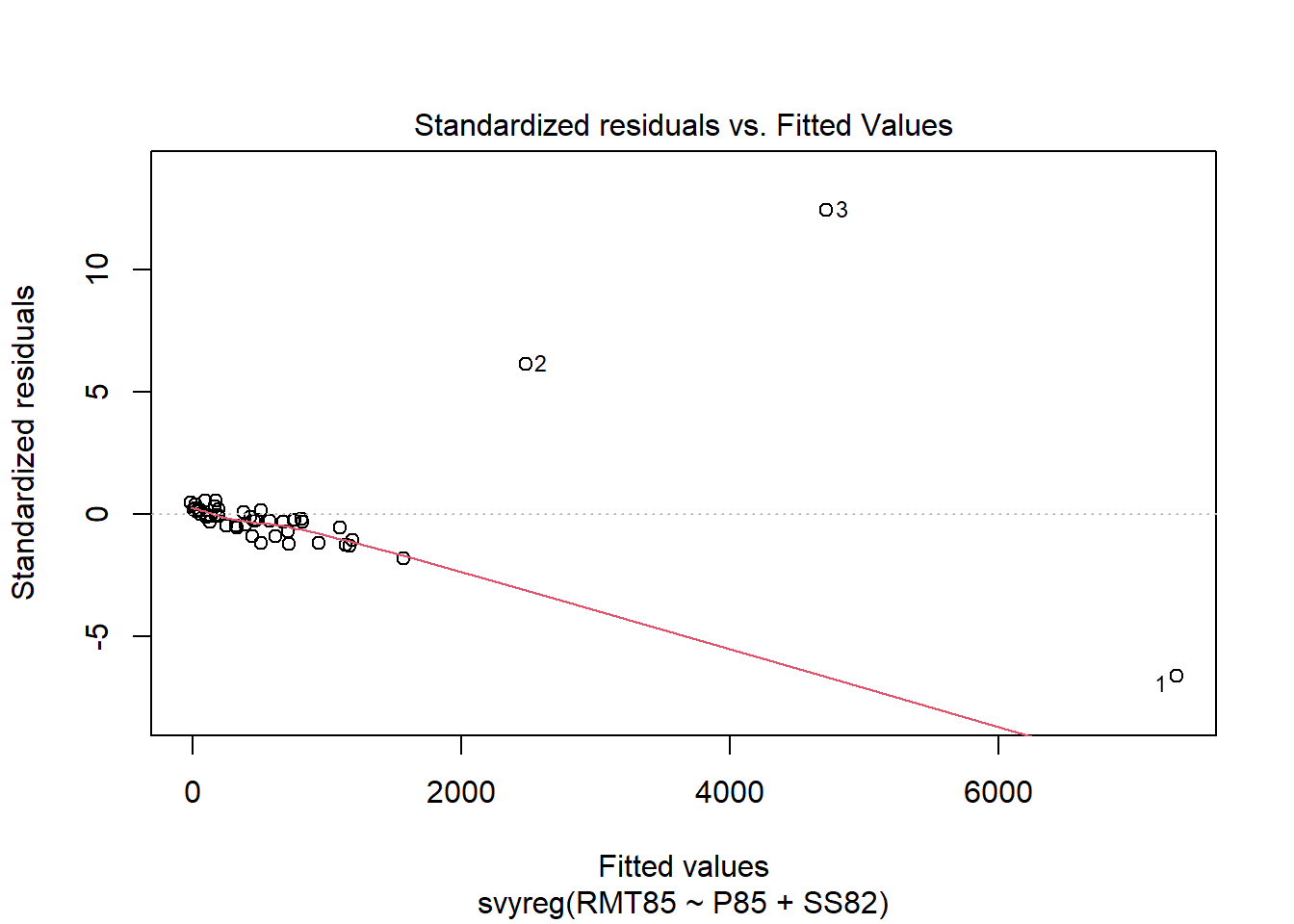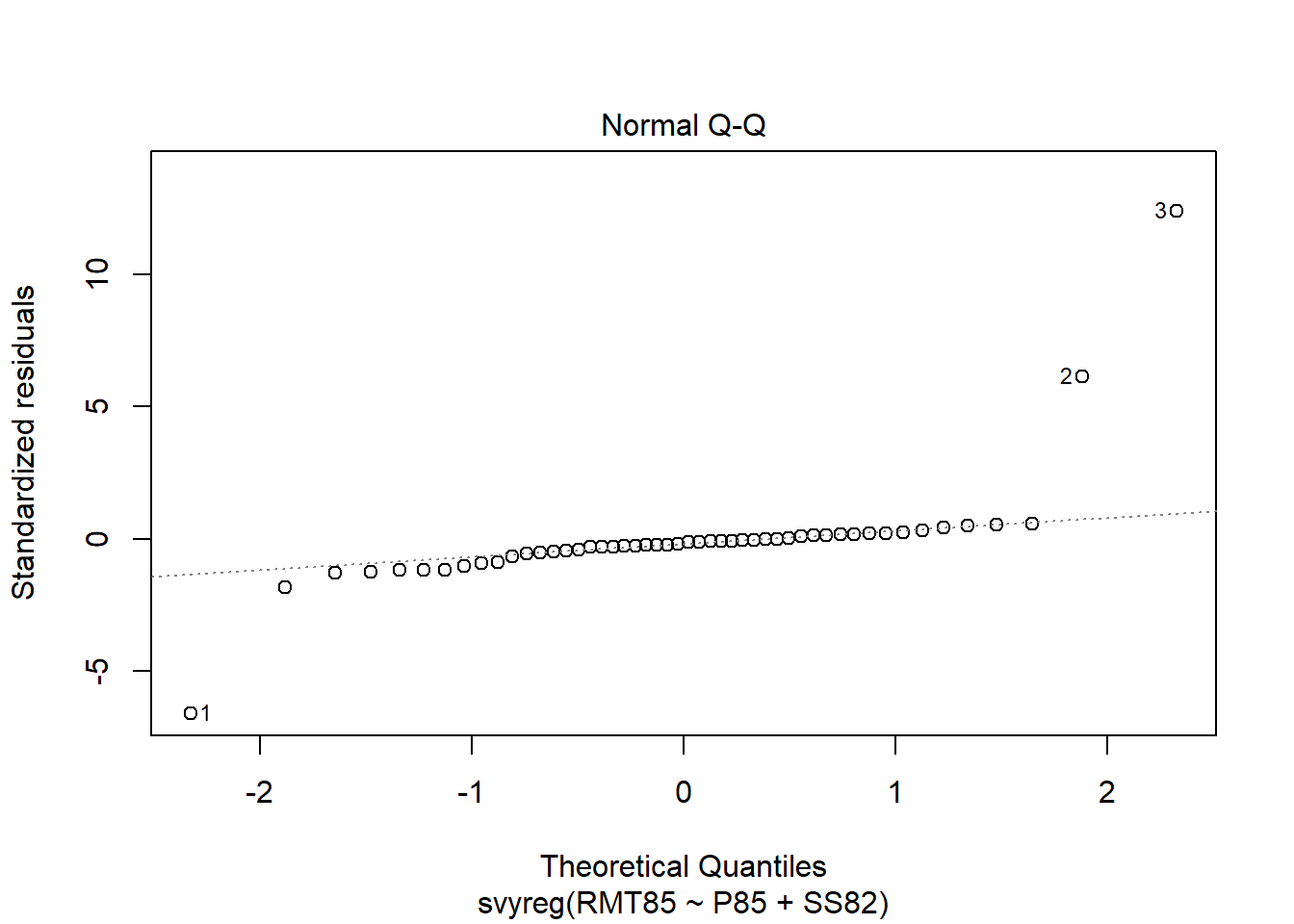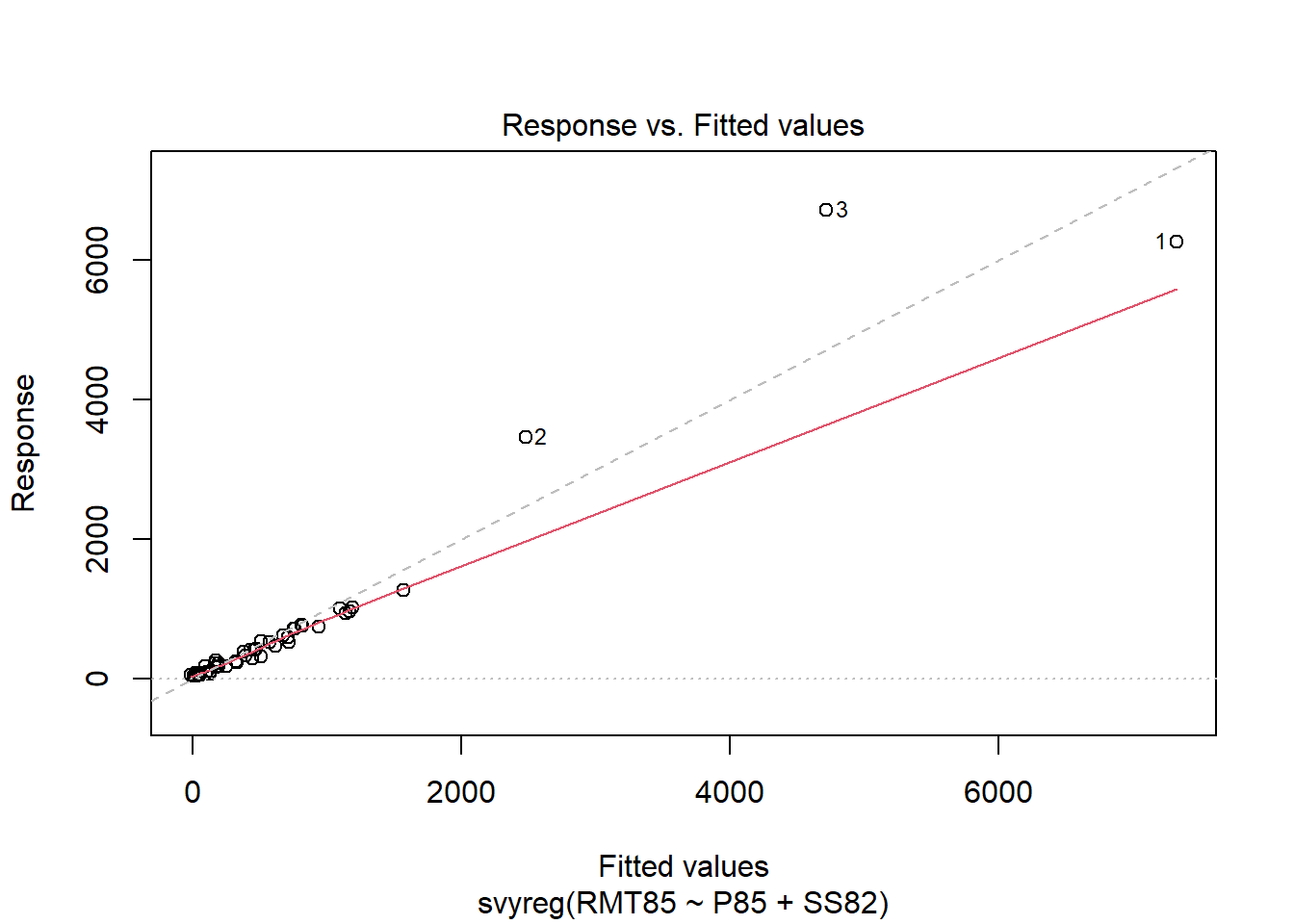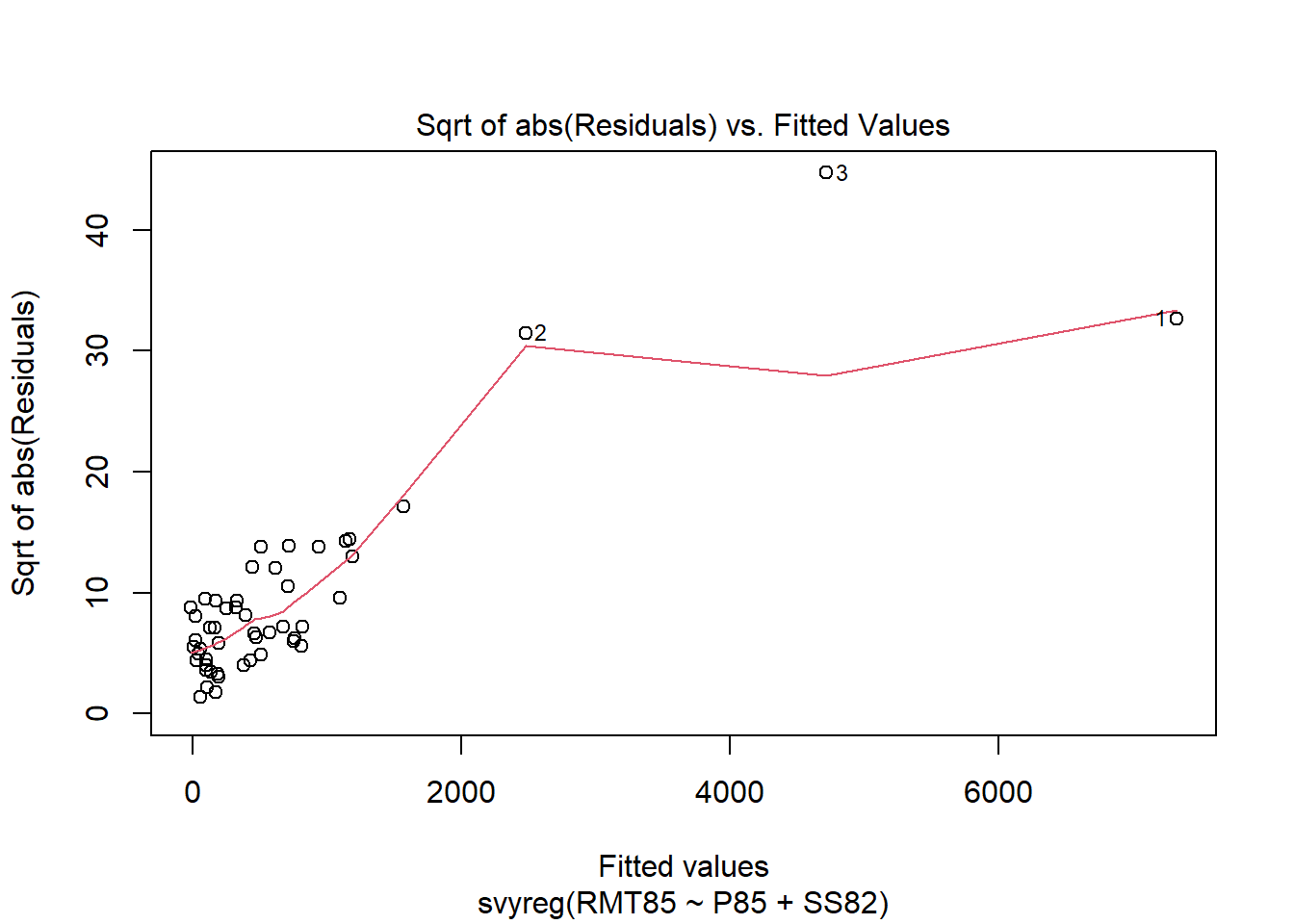Letting the issues aside, we want to predict the 1985 revenues total. The population totals of P85 and SS82 are known quantities (from registers) and are passed to the function svytotal_reg() via the totals argument. Because the model includes a regression intercept, we must also specify the population size N. The total is predicted using

> tot <- svytotal_reg(wls, totals = c(P85 = 8339, SS82 = 6301), N = 284, type = "ADU")
> tot
total   SE
RMT85 67914 2588

where type = "ADU" defines the “standard” GREG predictor, which is an asymptotically unbiased (ADU) estimator/ predictor, hence the name. By default, the argument check.names is set to TRUE in the call of svytotal_reg(). Thus, the names of arguments of totals are checked against the names of the estimated regression coefficients. If the names of totals are not specified, we call the function with check.names = FALSE. The estimated total, standard error and variance covariance can be extracted by the functions coef, SE(), and vcov().

The (estimated) approximate mean square error (MSE; which coincides with the estimated variance of the predictor) is

> mse(tot) / 1e6
 6.699817

The estimated total, standard error and variance covariance can be extracted by the functions coef, SE(), and vcov().

#### 3.2 Robust GREG

Consider the regression model from the last paragraph. Now, we compute a robust GREG predictor of the 1985 revenues total. The regression M-estimator with Tukey biweight (bisquare) $$\psi$$-function and the robustness tuning constant $$k=15$$ is

> rob <- svyreg_tukeyM(RMT85 ~ P85 + SS82, dn, k = 15)
> rob

Survey regression M-estimator (Tukey psi, k = 15)

Call:
svyreg_tukeyM(formula = RMT85 ~ P85 + SS82, design = dn, k = 15)

IRWLS converged in 6 iterations

Coefficients:
(Intercept)          P85         SS82
-13.6992       8.3275      -0.2038

Scale estimate: 16.46 (weighted MAD) 

The diagnostic plots look better than for the weighted LS estimator.

> plot(rob)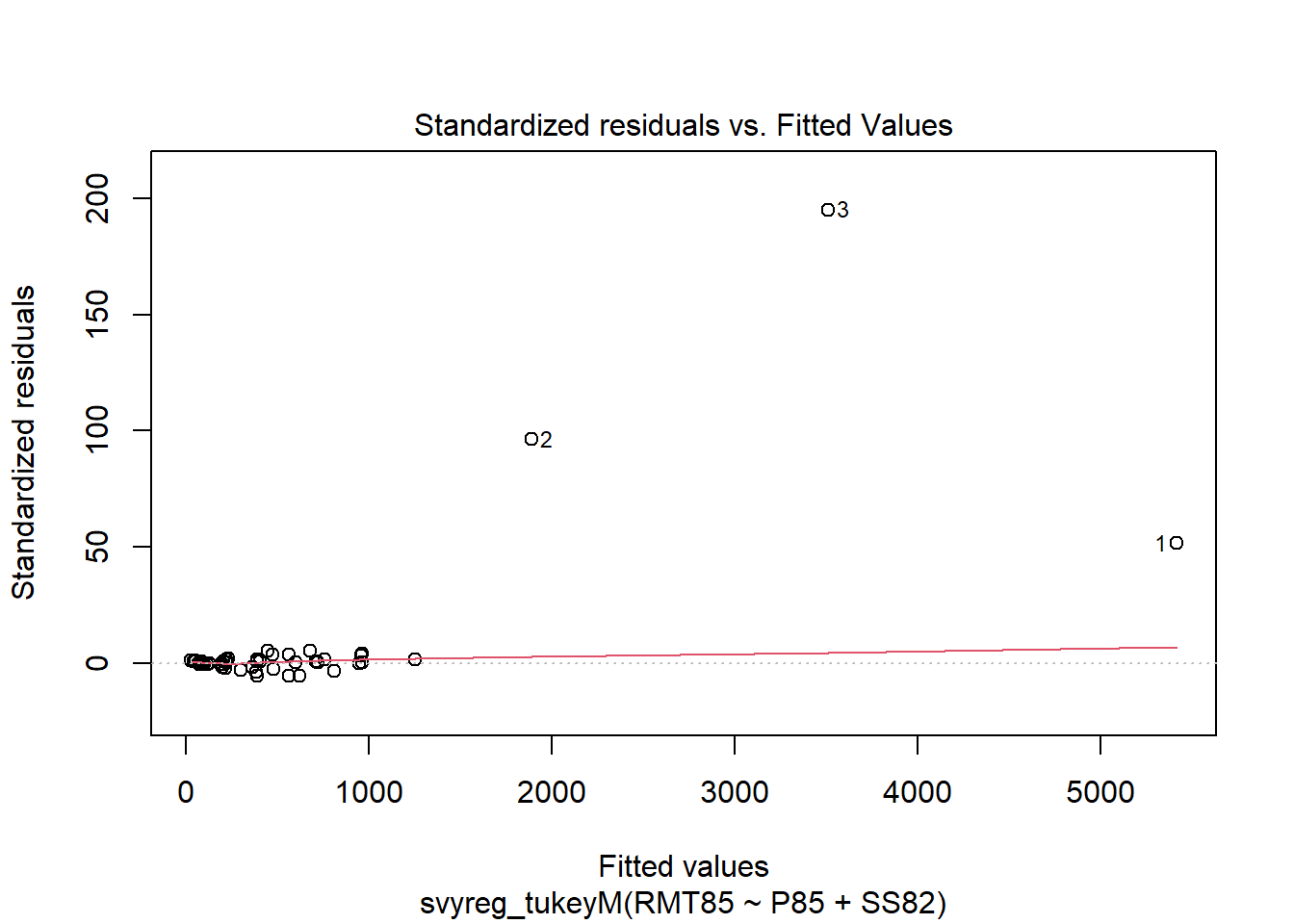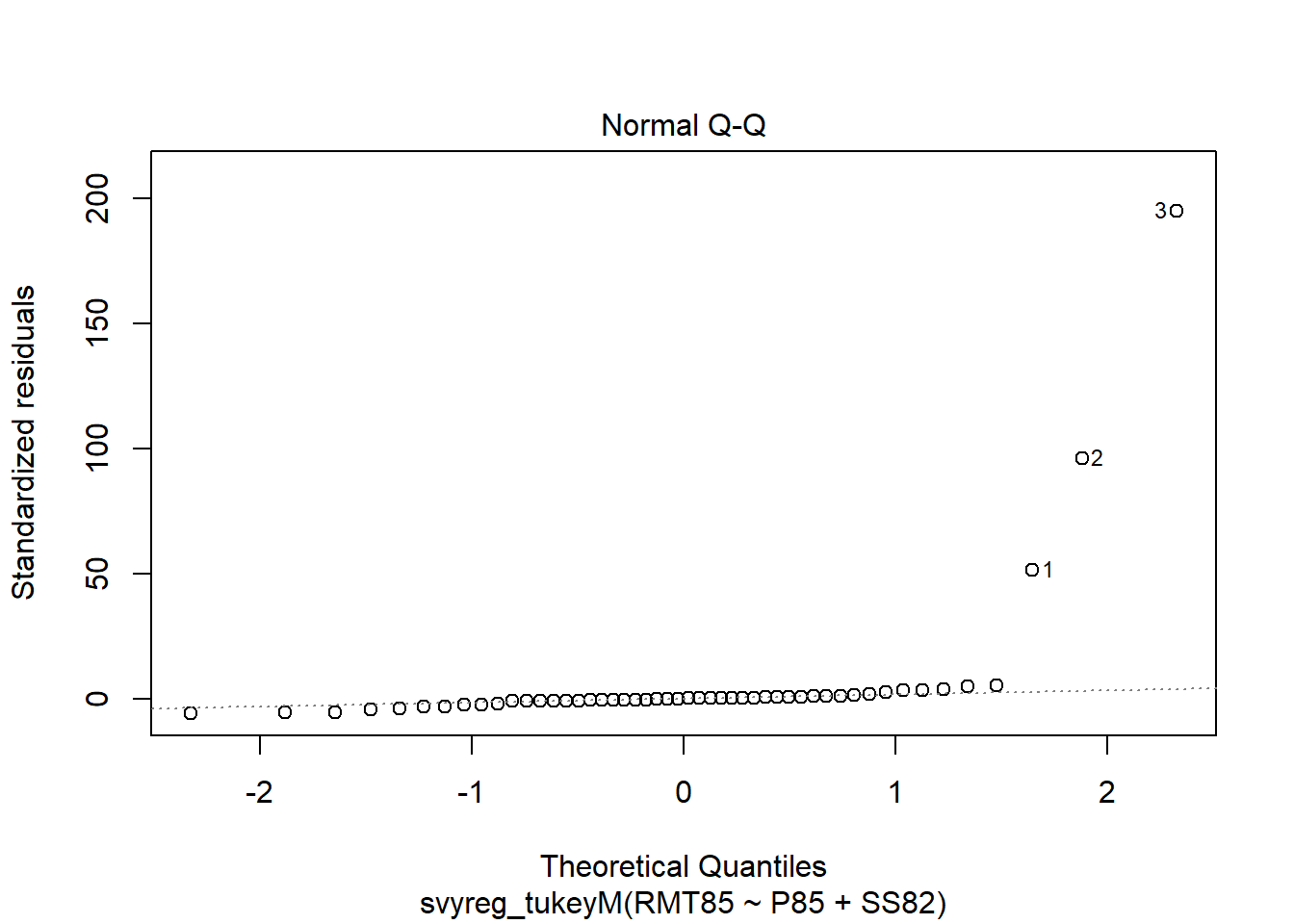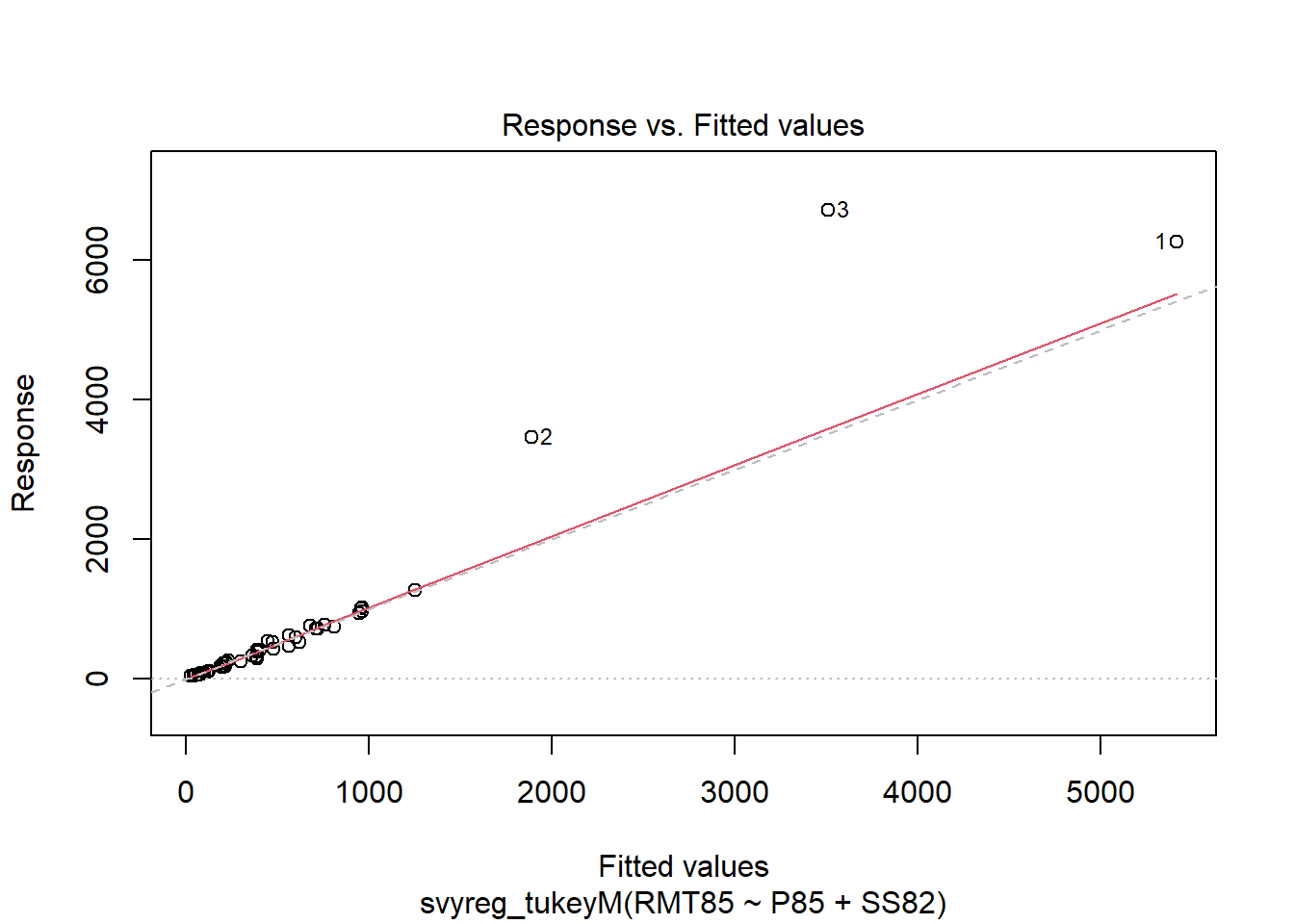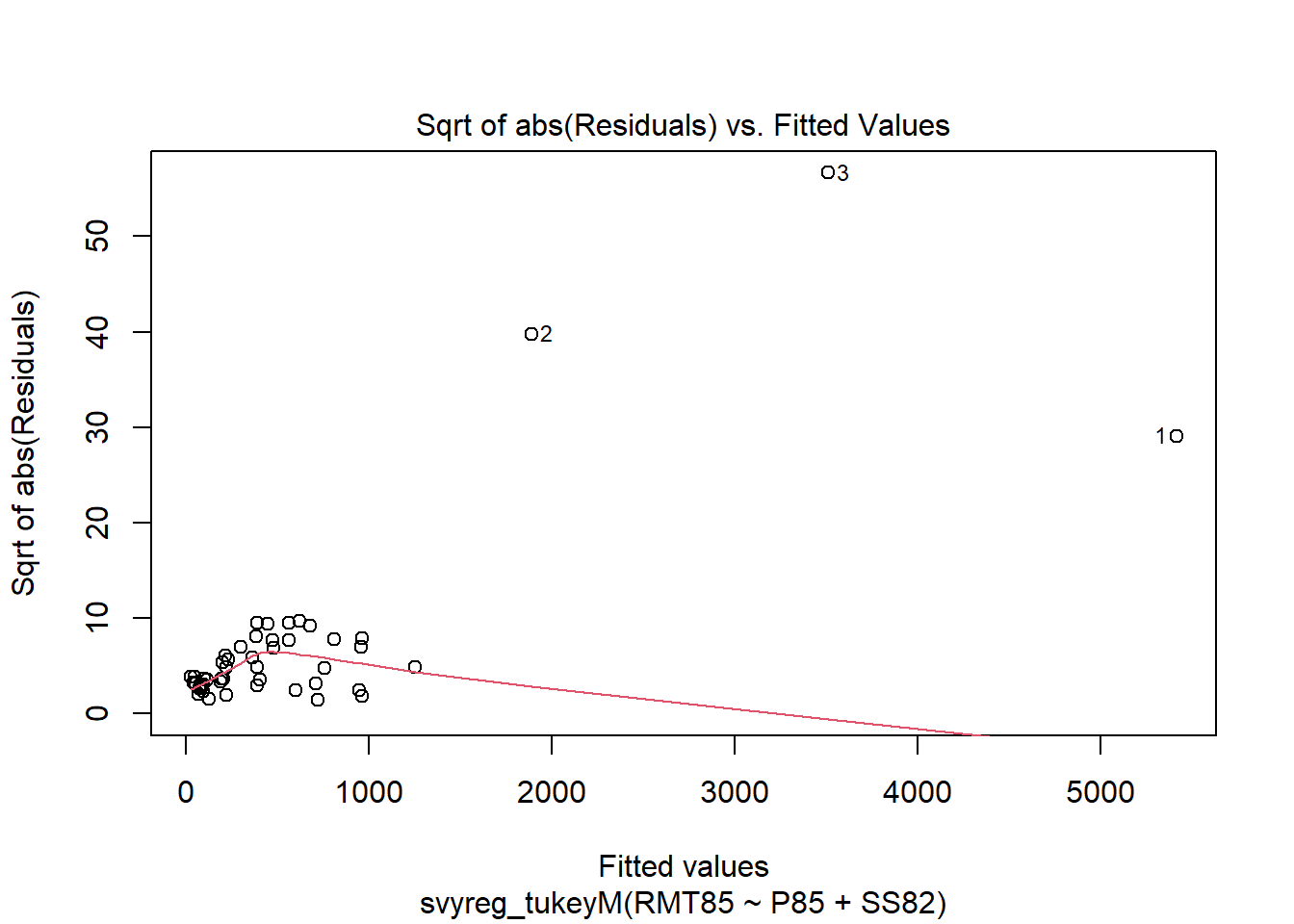The robust GREG predictor of the 1985 revenues total is

> tot <- svytotal_reg(rob, totals = c(P85 = 8339, SS82 = 6301), N = 284, type = "huber", k = 50)
> tot
total   SE
RMT85 66641 1287

where the prediction is based on the Huber $$\psi$$-function with tuning constant $$k = 50$$. The tuning constant for robust prediction should in general be larger than the one used for regression estimation. Observe that we have “mixed” the $$\psi$$-functions: Regression estimation based on the Tukey biweight $$\psi$$-function and prediction with the Huber $$\psi$$-function.

The (estimated) approximate MSE of the robust GREG predictor is

> mse(tot) / 1e6
 3.276374

which is considerably smaller than the MSE of the “standard” GREG. The estimated total, standard error and variance covariance can be extracted by the functions coef, SE(), and vcov().

See the help file of svymean_reg() or svytotal_reg() to learn more.

## References

LUMLEY, T. (2010). Complex Surveys: A Guide to Analysis Using R: A Guide to Analysis Using R, Hoboken (NJ): John Wiley & Sons.

LUMLEY, T. (2021). survey: analysis of complex survey samples. R package version 4.0, URL https://CRAN.R-project.org/package=survey.

SÄRNDAL, C.-E., SWENSSON, B. AND WRETMAN, J. (1992). Model Assisted Survey Sampling. New York: Springer-Verlag.

TILLE, Y. (2006). Sampling Algorithms. New York: Springer-Verlag.